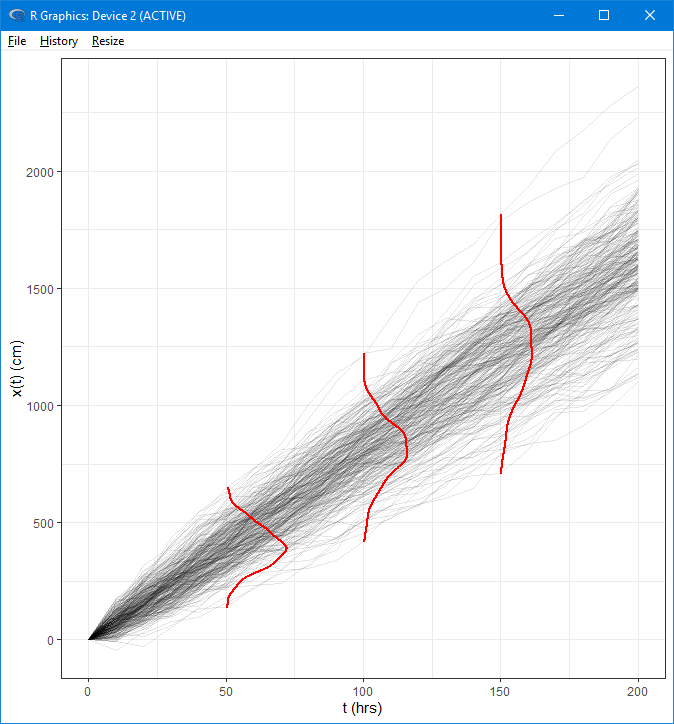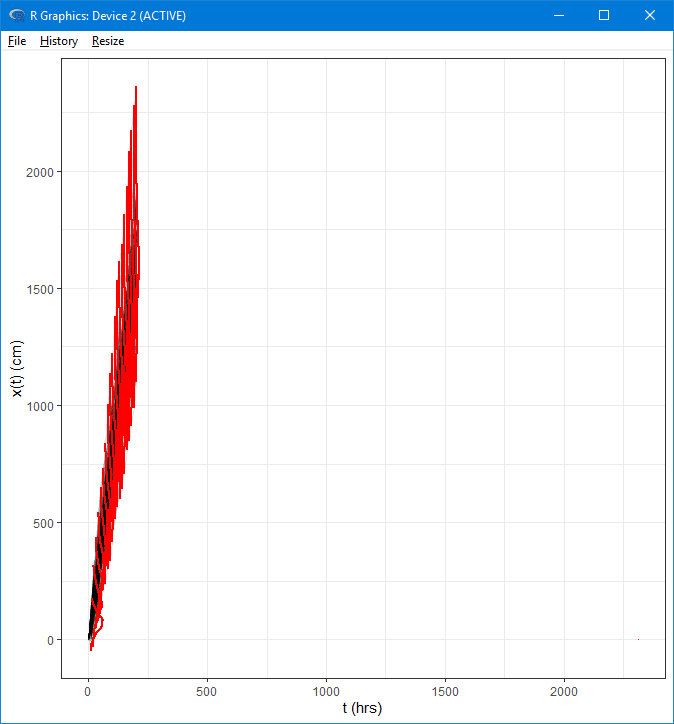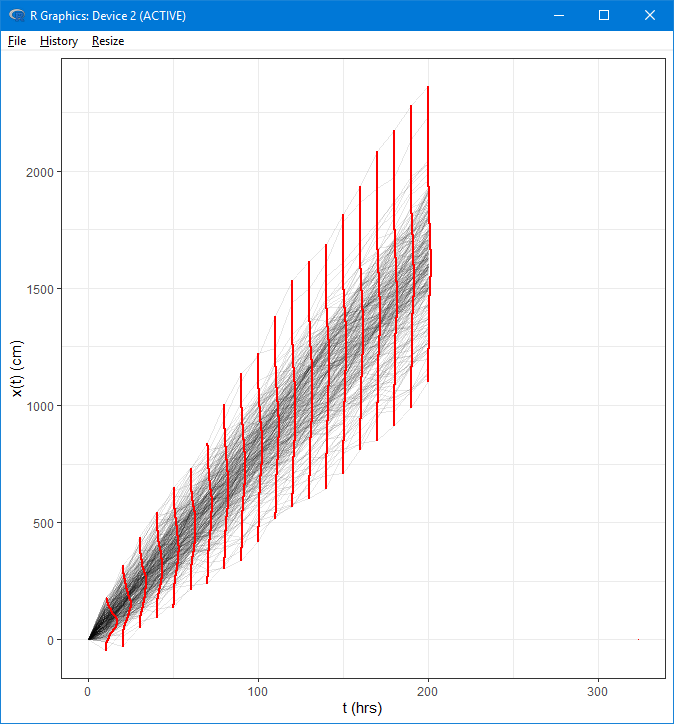# Funny behavior of argument `scale` in geom_vridgeline #47

Open
opened this issue Nov 18, 2019 · 1 comment

###mkoohafkan commented Nov 18, 2019 • edited
 Consider the following simulated data: ```library(tidyverse) library(ggridges) # markov chain parameters mu = 8 # cm/hr sigma = 4 # cm/sqrt(hr) x0 = 3 # initial condition tmax = 200 # end time deltat = 10 # time increment (hrs) reps = 300 # number of realizations random_walk = function() c(0, cumsum(mu*deltat + sigma*rnorm(n, sd = deltat))) + x0 # simulate random walks n = tmax/deltat res = cbind.data.frame(seq(0,tmax, by = deltat), replicate(reps, random_walk())) names(res) = c("time", paste("run", seq(1, ncol(res) - 1))) # format the data for plotting res.plot = gather(res, run, x, -time)``` I want to plot the distribution of values at select times as density ridgelines. This works perfectly with `geom_vridgeline` when a subset of the data is used: ```# extract specific times to compute marginal densities res.select = filter(res.plot, time %in% c(50, 100, 150)) ggplot(res.plot, aes(x = time, y = x, group = run)) + xlab("t (hrs)") + ylab("x(t) (cm)") + theme_bw() + # raw data geom_line(color = "black", alpha = 0.1) + geom_vridgeline(data = res.select, aes(group = time, width = ..density..), stat = "ydensity", scale = 5000, fill = NA, color = "red", size = 1)```However, it doesn't work so well when I try to this with the full dataset: ```ggplot(res.plot, aes(x = time, y = x, group = run)) + xlab("t (hrs)") + ylab("x(t) (cm)") + theme_bw() + # raw data geom_line(color = "black", alpha = 0.1) + geom_vridgeline(aes(group = time, width = ..density..), stat = "ydensity", scale = 5000, fill = NA, color = "red", size = 1)```The issue seems to be the `scale` argument which ends up expanding the y-axis. If I reduce the value of `scale`, the scale is expanded less but I lose the width of the ridglines: ```ggplot(res.plot, aes(x = time, y = x, group = run)) + xlab("t (hrs)") + ylab("x(t) (cm)") + theme_bw() + # raw data geom_line(color = "black", alpha = 0.1) + geom_vridgeline(aes(group = time, width = ..density..), stat = "ydensity", scale = 700, fill = NA, color = "red", size = 1)```Is this a bug, or am I misunderstanding something about the arguments?
###mkoohafkan commented Nov 24, 2019 • edited
 The issue turns out to be that for this dataset, all values of `x` at `time = 0` are 0, which results in an large (infinite?) value for the `ydensity` curve at time 0. However, the `ydensity` line for `time = 0` isn't plotted (because it's infinite?) so the issue isn't immediately obvious. Filtering the dataset for `time > 0` resolves the issue. Surprisingly, pull #21 doesn't resolve the issue.